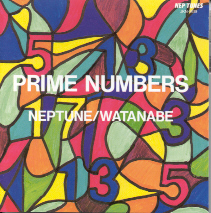# 17

This number is a prime.

Just showing those entries submitted by 'Teofilatto': (Click here to show all)Prime Numbers CD Image J. Neptune © 199417 is the smallest prime of form x^2 + y^2 + z, where x, y and z are three successive positive integers (2^2 + 3^2 + 4 = 17). [Teofilatto]